See how much you know about intervals in this quiz.

# Grade 3 - Intervals (Part 1)

An interval is the distance between two notes and the lower note of the interval in grade three is always the key-note of a scale that you have learned. Intervals were introduced in grade two and in grade three you need to be able to give the number of the interval and the type of interval.

Question 1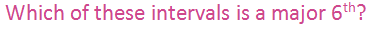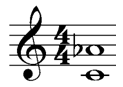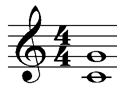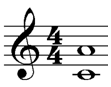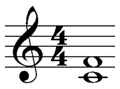Question 2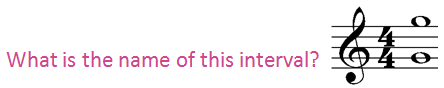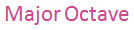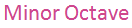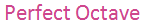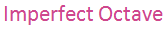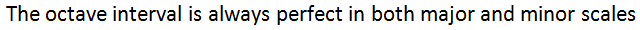Question 3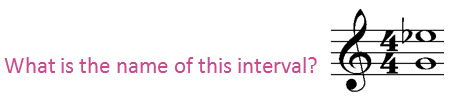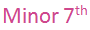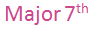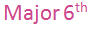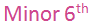Question 4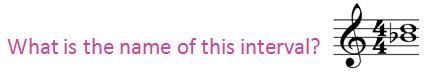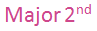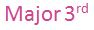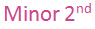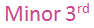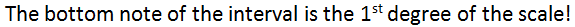Question 5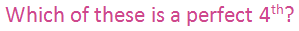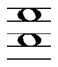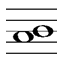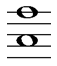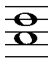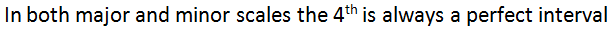Question 6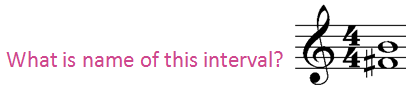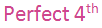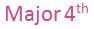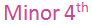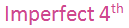Question 7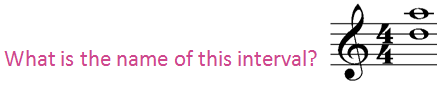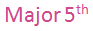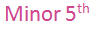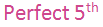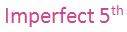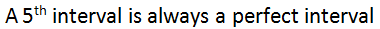Question 8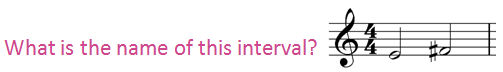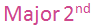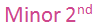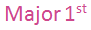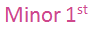Question 9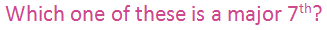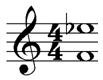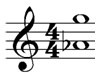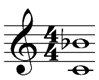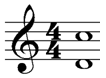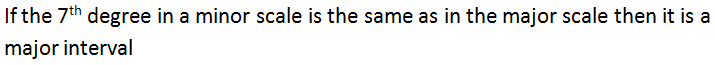Question 10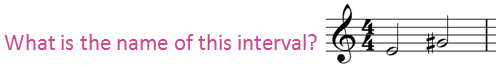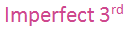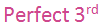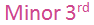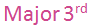Author:  Kathleen Shuster